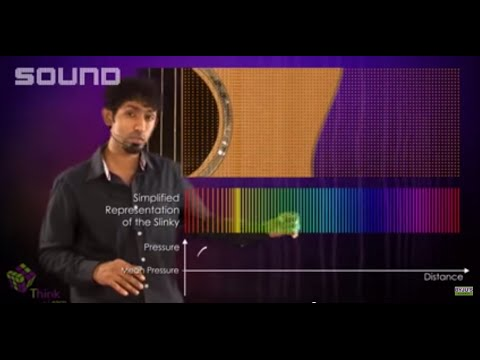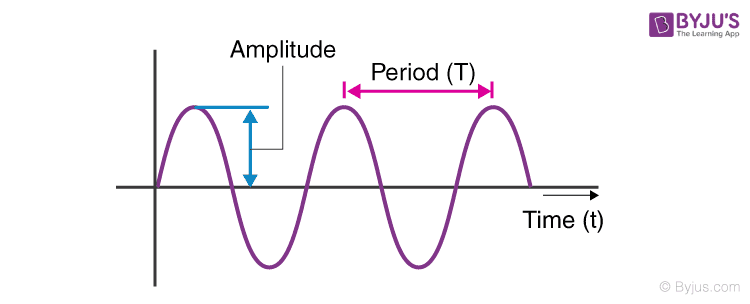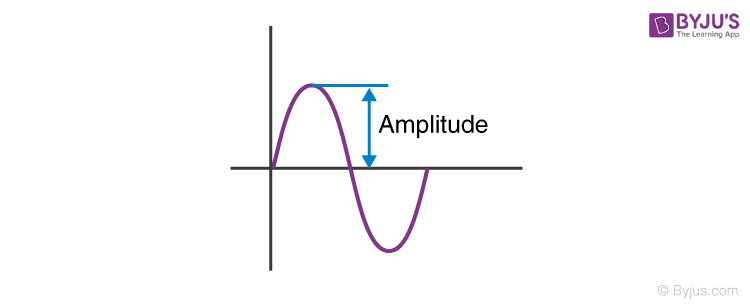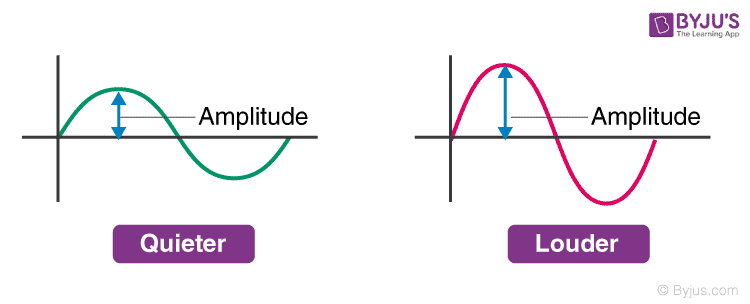# Amplitude Of A Wave

Sound is a form of energy that is produced by vibrating bodies. It requires a medium for its propagation. Hence, sound cannot propagate in a vacuum as there will be no material to transfer sound waves. The sound is produced by the back and forth motion of the object. This is known as sound vibration. It is also known as oscillatory motion. The regular rhythmic back and forth movement is referred to as the oscillation.
Table of ContentSome quantities that are used to explain sound are period, frequency and amplitude. They are described below:

## What is Period of Sound?

A period can be said to be the time taken to do something. If an event occurs repeatedly then the event is said to be periodic. The time taken by the periodic event to repeat itself is known as the period. The time taken by the particle to complete one vibration cycle is the time period for that particle.

 Period = 1/Frequency

## What is Frequency of Sound?

The number of oscillations per second is known as the frequency of oscillation. Its unit is hertz and is denoted by Hz. The frequency of a wave in general means how frequently the particles of a medium vibrate when a wave moves through the medium.

 Frequency = 1/Period## What is Amplitude of Sound?

The amplitude of a sound wave is the measure of the height of the wave. The amplitude of a sound wave can be defined as the loudness or the amount of maximum displacement of vibrating particles of the medium from their mean position when the sound is produced. It is the distance between crest or trough and the mean position of the wave.Loudness is directly proportional to the amplitude of the sound. If the amplitude of a sound wave is large, then the loudness of sound will be more. If the amplitude is small, then the sound will be feeble.### Characteristics of Sound Video

To better understand the above-explained concepts, watch the video given below.## Frequently Asked Questions – FAQs

### What is amplitude formula?

Following is the formula used for calculating the amplitude:
x = A sin($$\omega t+\phi$$)
Where,

• x is the displacement in metres
• A is the amplitude in metres
• $$\omega$$ is the angular frequency in radians/s
• t is the time in seconds
• $$\phi$$ is the phase shift in radians

### What is the wavelength of a wave with time period T travelling with a velocity V?

The wavelength of this wave would be the product of time period and velocity that is VT.

### Name a method that is used for the detection of ultrasonic waves which is produced in a medium.

Kundt’s tube is used for the detection of ultrasonic waves which is produced in a medium.

### In which direction does the air molecules move in the air if the sound wave travels from East to West?

East to West is the direction in which the air molecules move when the sound wave travels from East to West.

### What is the product of the time period and frequency of a wave?

The product of the time period and frequency of a wave is unity.

### What happens to the frequency of a wave if its time period increases?

The frequency decreases when the time period increases.

Stay tuned with BYJU’S for more such interesting articles.

Test your knowledge on Amplitude Frequency Period Sound